GeeksforGeeks App
Open AppBrowser
Continue

# Relative Minima and Maxima

Graphs are an integral part of calculus. They help us in analyzing the functions behavior and limits and lots of other things. We know graphs of some standard functions, but sometimes there are functions that are made up of a combination of these functions, and it is hard to predict their behavior. So we use derivatives and other things to locate their minima and maxima and then plot their graphs. Let’s see how to do that in detail.

### Maxima and Minima

Maximum and Minima are called critical points of the function. A maximum is a high point and a minimum is a low point. In a function, more than one maximum and minima points can exist. The points at which function attains the highest and lowest values are called global maximum and global minimum points.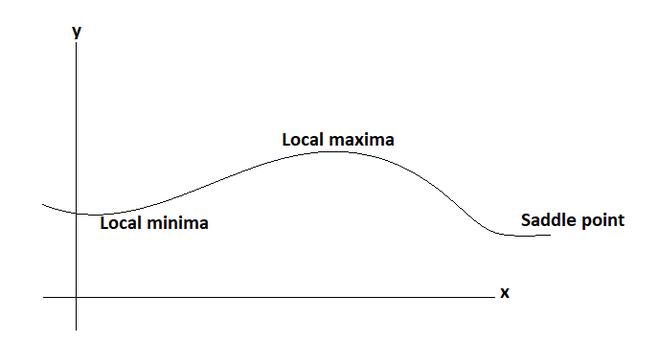How do we find these points?

In any smoothly changing function, the points where the function flattens out, give us either minima or maxima. Now, this statement gives rise to two questions.

1. How to recognize the points at which function flattens out?
2. Suppose we got a point at which function flattens i.e a critical point. How to tell whether it’s a minimum or a maximum?

To answer the first question let’s look at the slope of the function. The points where the function flattens out have zero slopes. We know that the derivative is nothing but the slope of the function at a particular point. So, we try to find the points where the derivative is zero.

f'(x) = 0

The solution to this equation gives us the position of the critical points. We still don’t know whether they are maxima or minima.

### Recognizing Maxima and Minima

As shown in the figure below, it can be seen that if the sign of the derivative is positive before the critical point and negative after the critical point, it is a maximum. Similarly, if it is negative before the critical point and positive after the critical point. It is a minimum. Maxima and minima can also be recognized by the second derivative test.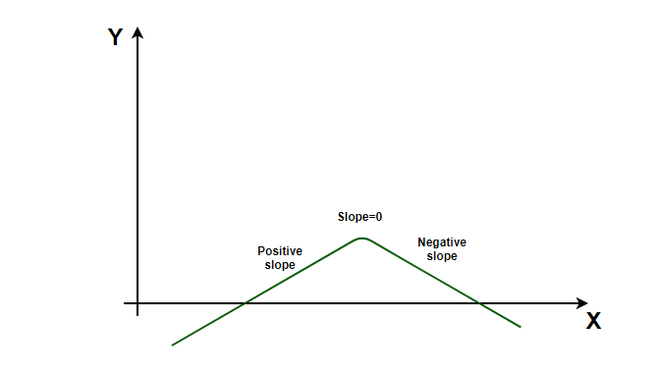Notice the figure carefully, and see that the slope of the curve is continuously decreasing, and then it becomes zero and goes further towards a negative value.

Second Derivative Test:

When a function’s slope is zero at x, then the second derivative f” at that point is:

1. f” < 0, if it is a maxima.
2. f” > 0, if it is a minima.

Note: If the second derivative is zero at the critical points, then the test fails.

### Analyzing a Function with its Derivative

Once, we have information about the minima and maxima of the function, we can start plotting a graph of that function. Let’s say we have a function f(x),

f(x) = x2 – 4

Step 1: Asymptomatic Values

Let’s see the asymptomatic values of the given function, that is the values this function takes at large positive and negative input. The function becomes infinite at large values of input.

Step 2: Find out the critical points

Putting f'(x) = 0 gives us the position of the critical points for the function. For the given function,

f'(x) = 0

⇒2x = 0

⇒ x = 0.

Thus, x = 0 is a critical point for this function. Now we need to find out whether it is a minimum or a maximum.

Step 3: Second Derivative Test

We use the second derivative test mentioned above to find out whether the given critical point is minima or maxima. In the above case,

f”(x) = 2

Notice that, f”(x) > 0. Thus, it must be a minimum.

Step 4: Value at critical points and roots.

Find the value of the function at critical points and find the roots if they exist. The value of the function at x = 0 also should be calculated. These things give us a fair idea about the shape of the graph.

f(0) = -4.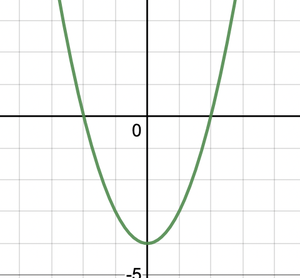Let’s see some examples on these concepts.

### Sample Problems

Question 1: Find all the critical points of the following function,

f(x) = x3 – 6x2 +11x – 6

Solution:

f(x) = x3 -6x2 +11x – 6

f'(x) = 3x2 -12x +11 = 0

⇒ 3x2 -12x +11 = 0

Using  Sridharacharya Formula,

x =x =x =x =x =Question 2: Plot the graph for f(x) = x + 4.

Solution:

Notice that as we increase the values of x on both positive and negative sides, the value of the function goes to positive and negative infinity.

Value of this function at x = 0.

f(0) = 4.

Now let’s look for the critical points.

f'(x) = 1.

There are no critical points, so there are no maxima or minima. Since the derivative is positive and constant, this function is strictly increasing at a constant rate. The constant derivative signifies that the function must be a line which cuts the y-axis at y = 4.

At last let’s look at the point at which the line cuts the x-axis. Putting f(x) =0

0 = x + 4

⇒x = -4

Now we got two points (-4,0) and (0,4). So, the curve will look like.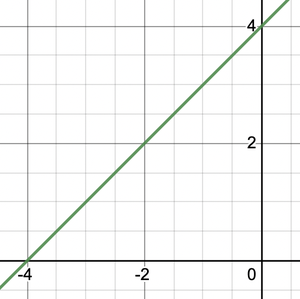Question 3: Given the function, f(x) = x2 – 4x + 4. Plot its graph.

Solution:

We will repeat the same steps for every function.

At x =0. f(x) = 4

Putting f(x) = 0 to find out where the curve intersects the x-axis.

x2 -4x + 4 = 0

⇒ x2 -2x -2x + 4 = 0

⇒ x(x – 2) -2(x – 2) = 0

⇒(x – 2)(x – 2) = 0

x = 2 is the point where the curve the x-axis.

We have both the points now,

f'(x) = 2x – 4  = 0

⇒x = 2

f”(x) = 2 > 0 thus x = 2 is a minima.

So, the graph of the function will look like,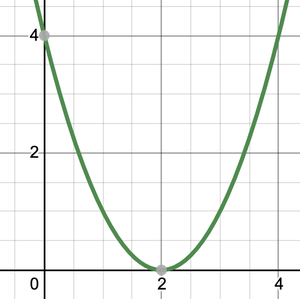Question 4: Given the function, f(x) =x2 -7x + 12. Plot its graph.

Solution:

at x = 0 , f(x) = 12.

Putting f(x) = 0,

x2 -7x + 12 =0

⇒ x2 -4x -3x + 12 = 0

⇒x(x – 4) -3(x – 4) = 0

⇒ (x -3) (x -4) = 0

Thus, x = 3 and x = 4 are the roots of this function.

Now let’s find out the critical points and their values.

f'(x) = 2x – 7 = 0d

⇒ x =f”(x) = 2 > 0 Thus, the critical point is minima.

So, the graph of this function will look like.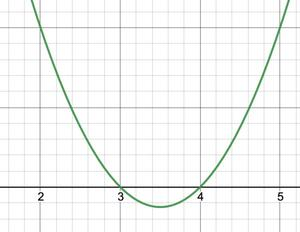Question 5: Given the function, f(x) =e + e-x . Plot its graph.

Solution:

The given function contains exponential.

At x = 0, f(0) = 2.

At x = ∞ or x = -∞, f(x) = ∞.

Finding out the critical points,

f'(x) = ex – e-x = 0

⇒ ex = e-x

Taking log both sides,

⇒ x = -x

⇒x = 0

Thus, x = 0 is a critical point.

f”(x) = ex + e-x > 0

Thus, x = 0 is a minimum.

f(0) = 2.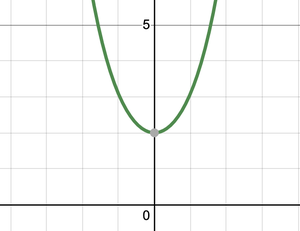My Personal Notes arrow_drop_up
Related Tutorials